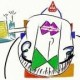The data visualization package lattice is part of the base R distribution, and like ggplot2 is built on Grid graphics engine. Deepayan Sarkar’s (the developer of lattice) book Lattice: Multivariate Data Visualization with R gives a detailed overview of how the package works. All the figures and code used to produce them is also available on the book website.

In order to give those interested an option to compare graphs produced by ggplot2 and lattice, I will attempt to recreate the book’s lattice graphs in ggplot2. There are 14 chapters in the book, so this means that there would be at least 13 more posts on the subject.

The output of both packages can be tweaked so that the graphs would look similar if not the same, however for the purposes of comparison, the standard settings (at least in ggplot2) are used when possible. The code used to create the images is in separate paragraphs, allowing easy comparison, and by clicking on the thumbnail, a bigger image file is also available.

## Chapter 1 – Introduction

Topics covered:

• Basic usage; high level functions
• Conditioning
• Superposition (a.k.a. grouping)
• “trellis” objects

### Figure 1.1

 ```> library(lattice) > library(ggplot2) > data(Chem97, package = "mlmRev")```

lattice

 ```> pl <- histogram(~gcsescore | factor(score), data = Chem97) > print(pl)```

ggplot2

 ```> pg <- ggplot(Chem97, aes(gcsescore)) + geom_histogram(binwidth = 0.5) + + facet_wrap(~score) > print(pg)```
 Note ggplot2 uses counts, not percentages by default.
 Note ggplot2 plots the facets starting from top-left, lattice starts from bottom-left.

### Figure 1.2

lattice

 ```> pl <- densityplot(~gcsescore | factor(score), data = Chem97, + plot.points = FALSE, ref = TRUE) > print(pl)```

ggplot2

 ```> pg <- ggplot(Chem97, aes(gcsescore)) + stat_density(geom = "path", + position = "identity") + facet_wrap(~score) > print(pg)```

### Figure 1.3

lattice

 ```> pl <- densityplot(~gcsescore, data = Chem97, groups = score, + plot.points = FALSE, ref = TRUE, auto.key = list(columns = 3)) > print(pl)```

ggplot2

 ```> pg <- ggplot(Chem97, aes(gcsescore)) + stat_density(geom = "path", + position = "identity", aes(colour = factor(score))) > print(pg)```
1.June 29, 2009 1:36 am

Awesome idea! Thanks for doing this.

2.June 29, 2009 2:05 am

I concur with Hadley. I’ve been doing most of my R graphics with the help of this book, so seeing the ggplot2 versions is a very good learning tool!

3.June 29, 2009 3:50 pm

+1 awesome!!!

4.August 8, 2009 11:45 pm

Much appreciate. This is very helpful.

5.June 14, 2010 10:24 pm

Thank you! this is brilliant

6.March 18, 2013 3:34 am

This series is excellent, thanks for making the effort.

7.May 5, 2015 2:32 pm

Hello.

Could you show an easy way to produce a scatter plot with error bars associated to each point, please?
From a dataframe with 4 columns: X, Y, errX, errY.
I’ve only seen complicated methods.

8.9.